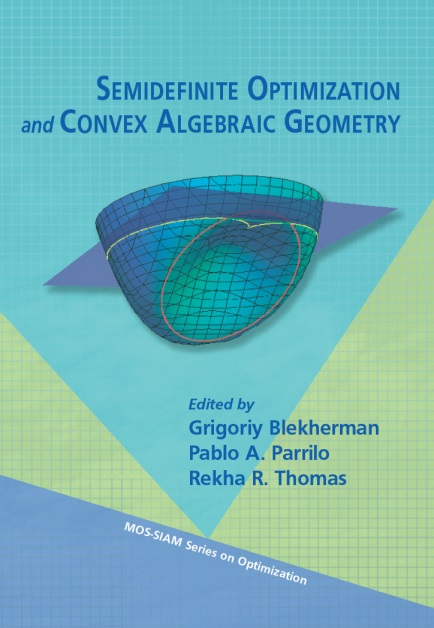# Semidefinite Optimization and Convex Algebraic GeometryThis book provides a self-contained, accessible introduction to the mathematical advances and challenges resulting from the use of semidefinite programming in polynomial optimization. This quickly evolving research area with contributions from the diverse fields of convex geometry, algebraic geometry, and optimization is known as convex algebraic geometry.

Each chapter addresses a fundamental aspect of convex algebraic geometry. The book begins with an introduction to nonnegative polynomials and sums of squares and their connections to semidefinite programming and quickly advances to several areas at the forefront of current research. These include semidefinite representability of convex sets, duality theory from the point of view of algebraic geometry, and nontraditional topics such as sums of squares of complex forms and noncommutative sums of squares polynomials.

Suitable for a class or seminar, with exercises aimed at teaching the topics to beginners, Semidefinite Optimization and Convex Algebraic Geometry serves as a point of entry into the subject for readers from multiple communities such as engineering, mathematics, and computer science. A guide to the necessary background material is available in the appendix.

```	    Publisher: SIAM
Series: MOS-SIAM Series on Optimization, Volume 13.
ISBN: 978-1-611972-28-3, 500 pages
Available: December 2012
```

#### Audience:

This book can serve as a textbook for graduate-level courses presenting the basic mathematics behind convex algebraic geometry and semidefinite optimization. Readers conducting research in these areas will discover open problems and potential research directions.

#### Contributors:

G. Blekherman (Georgia Tech), J. Gouveia (U. Coimbra), J.W. Helton (UC San Diego), I. Klep (U. Auckland), S. McCullough (U. Florida), J. Nie (UC San Diego), P. Parrilo (MIT). M. Putinar (UC Santa Barbara), P. Rostalski (U. Lübeck), B. Sturmfels (UC Berkeley), R. Thomas (U. Washington).

• Chapter 1: What is Convex Algebraic Geometry?
• Chapter 2: Semidefinite Optimization
• Chapter 3: Polynomial Optimization, Sums of Squares, and Applications
• Chapter 4: Nonnegative Polynomials and Sums of Squares
• Chapter 5: Dualities
• Chapter 6: Semidefinite Representability
• Chapter 7: Convex Hulls of Algebraic Sets
• Chapter 8: Free Convexity
• Chapter 9: Sums of Hermitian Squares: Old and New
• Appendix A: Background Material
• Index

Errata list